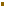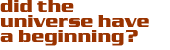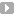### The Ten Commandments

Since we use Einstein's equation to study the origin of the universe, the ten non-linear partial differential equations contained in the Einstein equation can be regarded as the ten commandments of modern genesis.Following the ten commandments of modern genesis, we can study the early universe so long as we can reproduce the conditions of temperature, density, and pressure expected for the epoch in question. If we extrapolate the temperature-time relation from Einstein's equation to three minutes AB, we expect that the temperature was about 1,000,000,000 K. This is an enormous temperature, but because we can reproduce the same conditions in the laboratory, we have a good understanding of the behavior of matter in this extreme environment.

Earlier than three minutes AB the universe was so hot that nuclei could not exist. Just as any atom in the universe would have melted if the temperature was hotter than 3000K, nuclei melt at temperatures hotter than 1,000,000,000 K. Three minutes AB was the birth of the nuclear age; before then, the universe was made of the constituents of nuclei: neutrons and protons.

Our extrapolation back in time is rewarded, because we can predict what sort of nuclei were produced three minutes AB when neutrons and protons formed nuclei. Using what we learn from studying nuclear reactions in the laboratory, and using the modern laws of genesis to predict how the universe cooled as it expanded, we predict that three minutes AB the primordial neutrons and protons should form 75% hydrogen and 25% helium, with just a trace of lithium.Furthermore, the big-bang model predicts the abundance of different isotopes of hydrogen (normal hydrogen and heavy hydrogen) and helium (helium-4 and helium-3). The agreement between predictions and observations is an astounding success. The fact that the predictions agree so well with observations of the primordial elemental abundances gives us a lot of confidence that we understand the universe three minutes AB.

Contributed by: Dr. Edward Kolb

Cosmic QuestionsA Recipe for Primordial Soup

The Ten CommandmentsIntroductionTwo ThemesGrowing CosmologyThe Universe TodayInto the Primordial SoupThe Recipe for a UniverseThe Importance of NothingA Cosmic Symphony

#### Source:Rocky Kolb